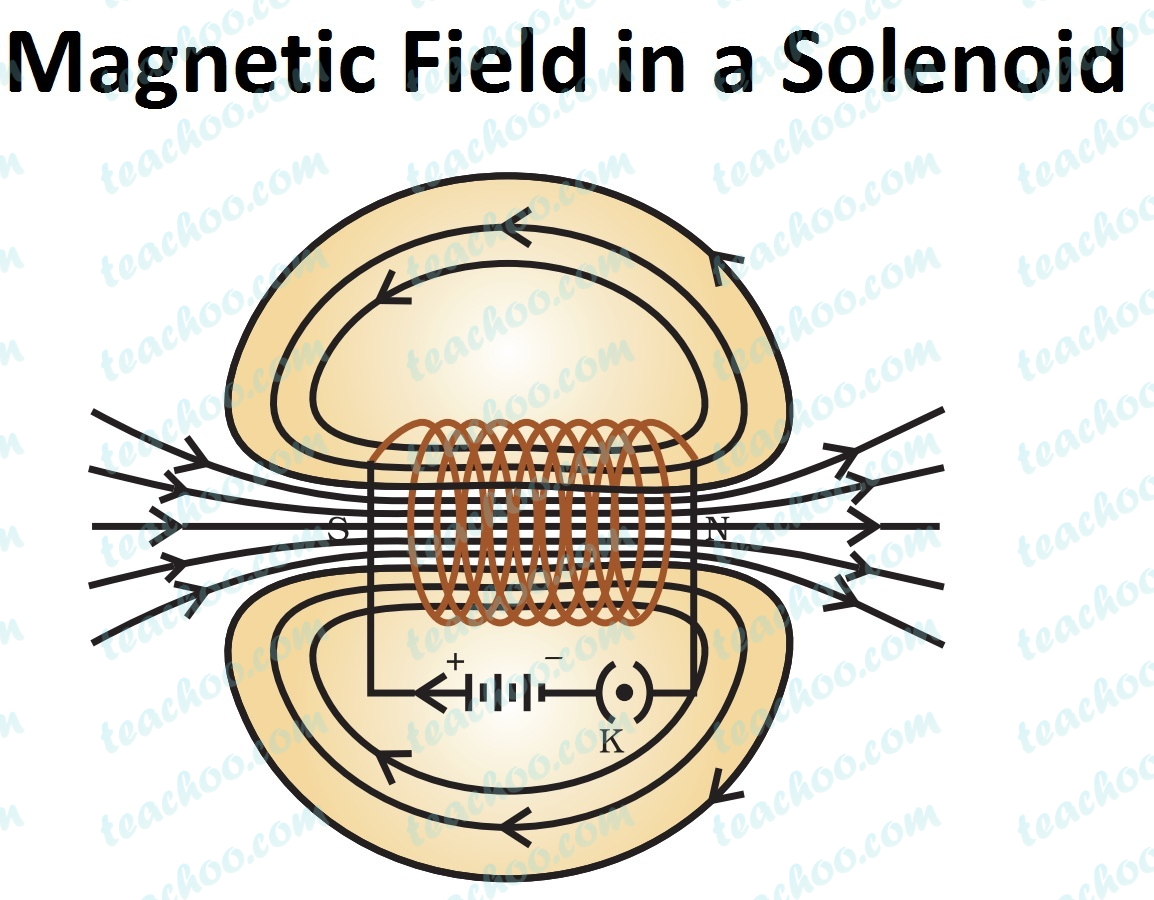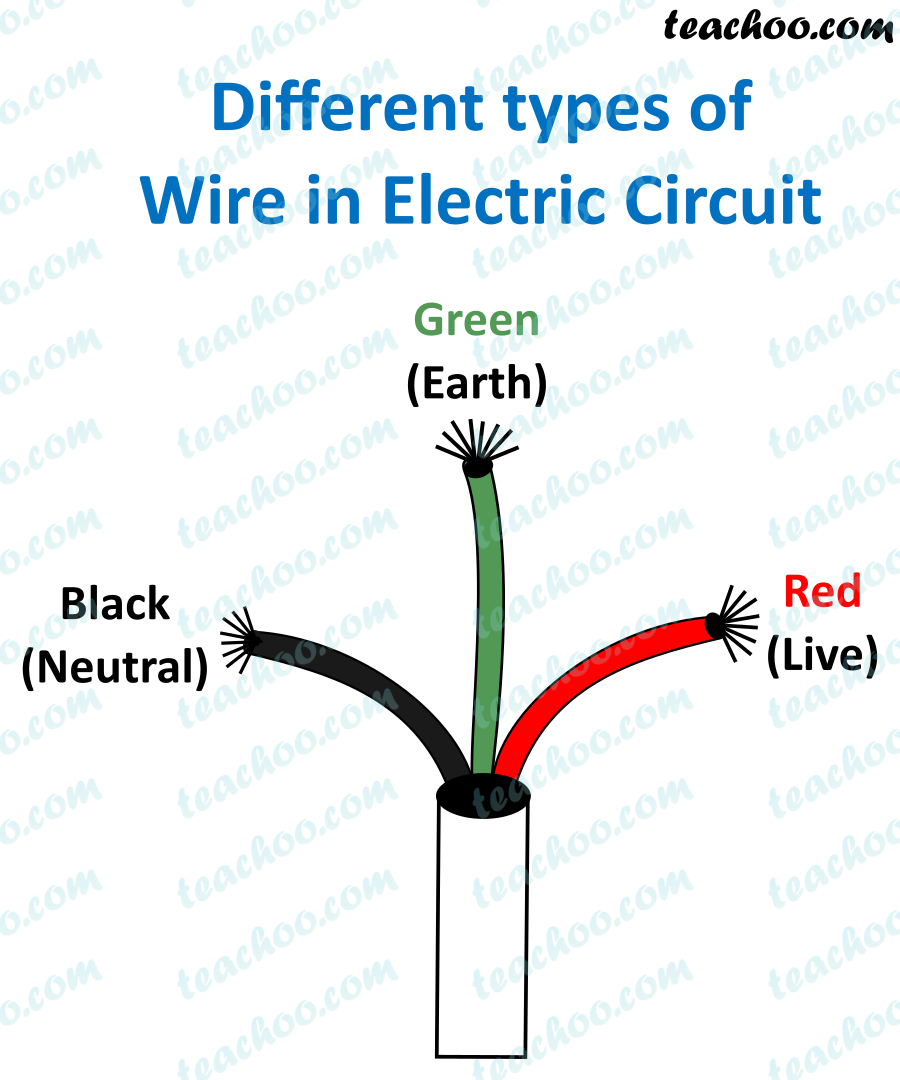NCERT Questions

Class 10
Chapter 13 Class 10 - Magnetic Effects of Electric Current (Term 2)

## State whether the following statements are true or false.

### (a) An electric motor converts mechanical energy into electrical energy.

False

Explanation : An electric motor converts electrical energy into mechanical energy.

### (b) An electric generator works on the principle of electromagnetic induction.

True

Explanation :

Electromagnetic induction is the process of production of electricity (electric current) when magnetic field changes

The electrical generator works on the principle that when a straight conductor is moved in a magnetic field, then current is induced in the conductor.

### (c) The field at the centre of a long circular coil carrying current will be parallel straight lines.

True

Explanation -

A long circular coil is a solenoid.

The field lines inside a solenoid are parallel.### (d) A wire with a green insulation is usually the live wire of an electric supply.

False

Explanation - A wire with a green insulation is usually the earth wire of an electric supply641 students have Teachoo Black. What are you waiting for?